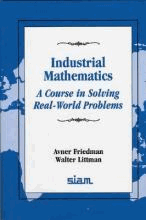# Industrial Mathematics : A Course in Solving Real-World Problems by Friedman, Avner

• R 250.00
Unit price per
Tax included.

ISBN: 9780898713244

Society for Industrial & Applied Mathematics,U.S. | 1 June 1993

Paperback | 150 pages

Industrial mathematics is a fast growing field within the mathematical sciences. It is characterized by the origin of the problems which it engages; they all come from industry: research and development, finances, and communications. The common feature running through this enterprise is the goal of gaining a better understanding of industrial models and processes through mathematical ideas and computations. The authors of this book have undertaken the approach of presenting real industrial problems and their mathematical modeling as a motivation for developing mathematical methods that are needed for solving the problems. With each chapter presenting one important problem that arises in today's industry, and then studying the problem by mathematical analysis and computation, this book introduces the reader to many new ideas and methods from ordinary and partial differential equations, and from integral equations and control theory. It brings the excitement of real industrial problems into the undergraduate mathematical curriculum.